## Pages

###Python Tutorial 4.0

Merging has ever been most haunting and confusing activity among all the data manipulation activities. At Ask Analytics we are committed to make data science "fun to learn" for you . We did make merging easy to learn in SAS as well as in R earlier, it is time to learn the subject in Python.

Practice more and more, as practice makes everyone perfect (not just man).
Merging in SAS :

Merging in R :

# Back to Python

import pandas as pData Age
Studentlist = ['Rajat','Vinod','Aarya','Vertika','Shobhit',]
Agelist=[25,28,22,23,30]
Age_Dictionary={ 'Student' : Studentlist ,'Age' : Agelist }
Data_Age=p.DataFrame(Age_Dictionary, columns=['Student','Age'])
Data_Age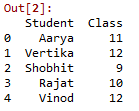Data Class

Studentlist = ['Aarya','Vertika','Shobhit','Rajat','Vinod']
Classlist=[11,12,9,10,12]
Class_Dictionary={ 'Student' : Studentlist ,'Class' : Classlist }
Data_Class=p.DataFrame(Class_Dictionary, columns=['Student','Class'])

Data_Class

# Let's now merge the two datasets on the matching key : students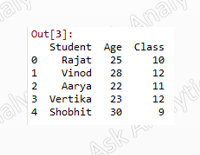Data_full

Data_full=p.merge(Data_Age,Data_Class,on='Student')

# In the above example, the matching key's values were common, now we are taking up a case where the matching key's values are not same across datasets.

Customeridlist = [1,2,3,4,5]Data_1
Productlist=['Metro','Metro','Bus','Bus','Bus']
Data1_Dictionary={ 'CustomerId' : Customeridlist ,'Product' : Productlist }
Data_1=p.DataFrame(Data1_Dictionary, columns=['CustomerId','Product'])
Data_1Data_2

Customeridlist = [2,4,6]
Citylist=['Delhi','Delhi','Mumbai']
Data2_Dictionary={ 'CustomerId' : Customeridlist ,'City' : Citylist }
Data_2=p.DataFrame(Data2_Dictionary, columns=['CustomerId','City'])
Data_2

### We shall now try 4 variants of the tables joins

# First full join : which is A union B i.e. All the components be it in A or B

Data_full=p.merge(Data_1,Data_2,on='CustomerId',how='outer')

# Inner join : Which is nothing but A intersection B i.e. components common in A and B

Data_inner=p.merge(Data_1,Data_2,on='CustomerId',how='inner')Inner Join

# Left join : Keeping A fixed i.e. All the components of A irrespective of that of B

Data_inner=p.merge(Data_1,Data_2,on='CustomerId',how='left')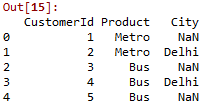Left Join

# Right join : Keeping B fixed i.e. All the components of B irrespective of that of A

Data_inner=p.merge(Data_1,Data_2,on='CustomerId',how='right')Right Join

###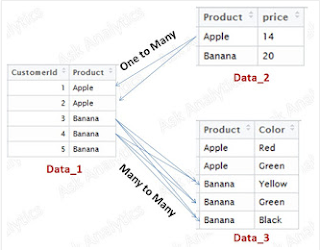One to many and many to many joins

For understanding the one to many (same as "many to one") and many to many merging, let's prepare three datasets:

Customeridlist = [1,2,3,4,5]Data_1
Productlist=['Apple','Apple','Banana','Banana','Banana']
Data1_Dictionary={ 'CustomerId' : Customeridlist ,'Product' : Productlist }
Data_1=p.DataFrame(Data1_Dictionary, columns=['CustomerId','Product'])
Data_1

Productlist=['Apple','Banana']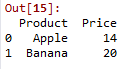Data_2
Pricelist = [14,20]
Data2_Dictionary={'Product' : Productlist ,  'Price' Pricelist }
Data_2=p.DataFrame(Data2_Dictionary, columns=['Product', 'Price'])
Data_2Data_3
Productlist=['Apple','Apple','Banana','Banana','Banana']
Colorlist=['Red','Green','Yellow','Green','Black']
Data3_Dictionary={ 'Product' : Productlist, 'Color' : Colorlist}
Data_3=p.DataFrame(Data3_Dictionary, columns=['Product','Color'])
Data_3

Let's first do a one to many merge

Data_one_to_many=p.merge(Data_1,Data_2,on='Product')

Output:

And now it's time for doing a many to many merge

Try_many_to_many = p.merge(Data_1,Data_3,on = 'Product')

Output :

# You can see , in case on many to many join, it makes a Cartesian product, similar to SQL join.

### Last lesson of merging

Imagine, if same set of information (same column across datasets) is there in the the datasets, how would python merge these. Would the column bearing same name as in another dataset would replace it ?

Let's see !

rulingpartylist=['SP','BJP','BJP','BJP']
Datax_dictionary={'State':statelist,'ruling_party':rulingpartylist}
Data_x=p.DataFrame(Datax_dictionary,columns=['State','ruling_party'])
Data_x

Datay_dictionary={'State':statelist,'ruling_party':rulingpartylist,'language':languagelist}
Data_y=p.DataFrame(Datay_dictionary,columns=['State','ruling_party','language'])
Data_y

p.merge(Data_x,Data_y,on='State')

Merging based on multiple keysProduct_Sales_List = {

'Product'   : ['A','A','B','B'],
'Year'      : [2014,2015,2014,2015],
'Sale'      : [500,700,1100,1450]      }

Product_Sales=p.DataFrame(Product_Sales_List,columns=['Product','Year','Sale'])

Product_Sales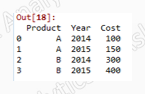Product_Cost_List = {

'Product'   : ['A','A','B','B'],
'Year'      : [2014,2015,2014,2015],
'Cost'      : [100,150,300,400]      }

Product_Cost=p.DataFrame(Product_Cost_List,columns=['Product','Year','Cost'])

Product_Cost

p.merge(Product_Sales,Product_Cost,on=['Product','Year'])

Enjoy reading our other articles and stay tuned with us.

Kindly do provide your feedback in the 'Comments' Section and share as much as possible.